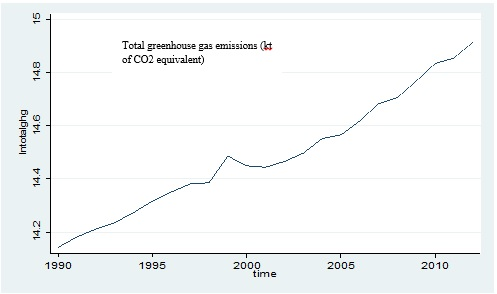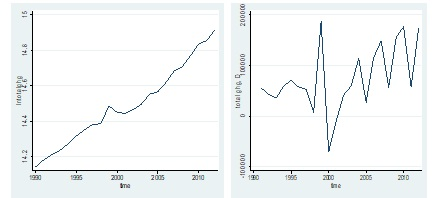# Establishing a relationship between FDI and air pollution in India

The previous articles in this study established how Foreign Direct Investment (FDI) affects the economic growth of a country. In India, in the last two decades, the inflow of FDI has grown significantly. Similarly, its environmental pollution has also been rising since 1991 due to an increase in economic activity. This article empirically investigates the impact of FDI on air pollution in India. In order to do so, it establishes a long run association between FDI inflows and air pollution in the post reform period, i.e. since 1991. The previous article identified the common air pollution indicators, of which Greenhouse Gas (GHG) emissions is the most significant. The figure below shows that total GHG emissions have increased drastically in India.Figure 1: Total GHG in India during 1990-2010 (source: World Bank)

## A time series study to establish a relation between FDI & GHG emissions and air pollution

The annual time series data for FDI, total GHG emissions (kt of CO2 equivalent) and other GHG emissions of hydrofluorocarbons (HFCs), perfluorocarbons (PFCs) and sulphur hexafluoride (SF6) (thousand metric tons of CO2 equivalent) were extracted from World Bank for the period 1990 to 2012. The reason for taking two data series for GHG is the separate reporting of them in the World Bank database.

For the regression analysis, there are two equations. All the variables in the equations are present in the natural log form as it shows the impact in terms of percentage rather than units. ‘ln(FDI)’ is the independent variable in the model. ‘ln(total GHGs)’ and ‘ln(other GHGs)’ are the dependent variables. The following equations show the relationship between the variables.

Total GHGs and other GHGs are measured as a function of FDI.

##### Variables
Unit root test To check stationarity in the data Ln(FDI), ln(total GHGs), ln(other GHGs)
Johansen cointegration test To check the long-run relationship Ln(FDI), ln(total GHGs), ln(other GHGs)
Time series regression To determine the impact of FDI on air pollutants Ln(FDI), ln(total GHGs), ln(other GHGs)

Table 1: Tests applied for estimating the impact of FDI on air pollution in India

## The procedure of the time series analysis

The first step in the empirical analysis is to check stationarity of data by applying Augmented Dickey-fuller (ADF) test and Phillips-Perron test. Stationarity in time series will have no predictable patterns in the long-term. On the contrary, a non-stationary time series includes trends and seasonality effect and gives spurious results in regression, which leads to poor understanding and forecasting. Therefore, stationarity is important for better results with constant mean, variance and covariance over time. Differencing helps make a series stationary which will transform a series into a completely random one.

Furthermore, to check the validity of the long run relationship between the dependent and the independent variables, the Johansen co-integration test is suitable. When the dependent and independent variables are non-stationary at a level, it means that the variables are co-integrated. As the figures 2, 3 and 4 below show, the series are non-stationary at level. However, after differencing, the series become stationary. STATA software is used to interpret the long-term impact of FDI on GHGs.

Hence the equations above showing the regression is not a spurious one, even though individually ‘ln(total GHGs)’ and ‘ln(FDI)’ are non-stationary at the level. Thus, there is co-integration among them, i.e., there exists a stable long-run relationship between total GHG emissions and growth in FDI inflow during 1990-2012.Figure 2: Trend of ln(FDI) and stationarity of D.ln(FDI)Fig 3: Trend of ln (total GHGs) and stationarity of D.ln(total GHGs)Fig 4: Trend of ln(other GHGs) and stationarity of D.ln(other GHGs)

## Unit root test

The null and alternative hypotheses for the ADF and Phillips-Perron test are the following:

• H0= Series is a non-stationary i.e. presence of unit root.
• H1= Series is stationary i.e. no unit root.

The criteria state that if PP and ADF t-statistics are greater than the critical values, then reject the null hypothesis. Therefore the series is stationary. On the other hand, if t-statistics is less than the critical values, then do not reject the null hypothesis.

PP  t-
statistic
##### PP at 5% Level
Total greenhouse gas emissions 1.153 -3.750 -3.000 0.751 -3.750 -3.000
ΔTotal greenhouse gas emissions -5.514 -3.750* -3.000* -5.515 -3.750* -3.000*
FDI -1.312 -3.750 -3.000 -1.316 -3.750 -3.000
ΔFDI -5.420 -3.750* -3.000* -5.672 -3.750* -3.000*
Other greenhouse gas emissions -1.985 -3.750 -3.000 -2.126 -3.750 -3.000
ΔOther greenhouse gas emissions -6.813 -3.750* -3.000* -5.928 -3.750* -3.000*

Table 2: Unit root test results for examining the impact of FDI on air pollution in India

The table above shows that all the variables are non-stationary at level. The variables become stationary after first differencing, so investigate the long-run relationship among them. Therefore the null hypothesis of the existence of unit root or non-stationarity in the data can be rejected at the first difference.

### Co-integration test

Johansen co-integration test shows the long run association between the variables. When the variables are co-integrated, it means that they have a long run equilibrium relationship.

#### 5% Critical Value

0 32.2461 29.68 18.9464 20.97
1 13.2997* 15.41 11.1744* 14.07

Table 3: Johansen Co-integration Test (Trace and Max Value stat) results for FDI and air pollution in India

This test assumes the maximum likelihood method and gives two statistics: eigenvalue statistic and maximum statistic. The above results of the Johansen co-integration test imply that there is one co-integration between the variables. The results of both trace and max statistic suggest that there is a long run association between FDI and GHG.  The findings are in line with Kumar & Chander, (2016), showing a long run relationship between FDI inflow and carbon dioxide emissions in India between 1981 and 2011 as it is one of the most abundant GHG in the atmosphere.

### Regression analysis

The log-log regression model helps to measure the impact of FDI on GHGs.

ln(total GHGst)= β0 + β1ln(FDIt) + ut

The null and alternative hypotheses are as follows:

• H0= FDI has no impact on total GHGs or other GHGs
• H1= FDI impacts total GHGs or other GHGs
 ln (total GHGs) Coefficient t-value P-value R2 ln(FDI) 0.1188636*** 11.37 0.000 0.8603 Cons 11.86443*** 51.19 0.000

Table 4:  Regression results for impact of FDI on total GHG emissions causing air pollution in India
(Note: Superscripts “*” denote 1% and ““5% significance levels)

The table above presents the regression results between ‘ln(total GHGs)’ and ‘ln(FDI)’. The results reveal that an increase in FDI by 1% will raise total GHG emissions by 0.11%. The coefficient of FDI is positive and significant at 5% level.

To measure the impact of FDI on other GHGs, the equation is:

ln(other GHGst)= β0 + β1ln(FDIt) + ut

 ln(other GHGs) Coefficient t-value P-value R2 ln(FDI) 0.1402354** 2.49 0.021 0.2284 Cons 7.855737*** 6.30 0.000

Table 5: Regression results for the impact of FDI on other GHG emissions causing air pollution in India
(Note: Superscripts “*” denote 1% and ““5% significance)

Table 5 shows that there is a positive significant impact of FDI on other GHG emissions. Therefore, the higher the level of FDI, higher will be the emission of the GHGs of HFC, PFC and SF6.  The estimated coefficient reveals that a 1% increase in FDI will lead to a 0.14% increase in these other GHGs. Similar results have been found by (Acharyya, 2009; Zheng & Sheng, 2017) with respect to the significant impact of  FDI on carbon dioxide emissions in India and China respectively.

## Positive and significant impact of FDI on GHG emissions

The analysis in this article finds the evidence of a positive and significant effect of FDI on GHG emissions thus indicating higher air pollution in India during 1990-2012. The results of co-integration tests show that there is a significant long-term association between GHG emissions and FDI.  However, it needs to be pointed out that there are several other factors too which contribute to air pollution positively such as population growth, deforestation, urbanization, and human activities. There are several other indicators of air pollution. Therefore the next article shows the analysis for impact of FDI on pH values of India, one such indicator of air pollution.

### Rashmi Sajwan

Research Analyst at Project Guru
Rashmi has completed her bachelors in Economic (hons.) from Delhi University and Masters in economics from Guru Gobind Singh Indrapastha University. She has good understanding of statistical softwares like STATA, SPSS and E-views. She worked as a Research Intern at CIMMYT international maize and wheat improvement centre. She has an analytical mind and can spend her whole day on data analysis. Being a poetry lover, she likes to write and read poems. In her spare time, she loves to do Dance.

#### Latest posts by Rashmi Sajwan (see all)We are looking for candidates who have completed their master's degree or Ph.D. Click here to know more about our vacancies.